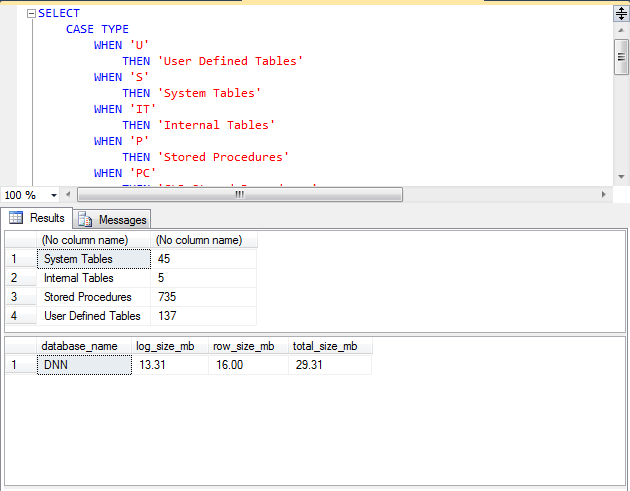```DELETE FROM [dbo].[EventLog];
-- or
TRUNCATE TABLE [dbo].[EventLog];
DELETE FROM [dbo].[SiteLog];
-- or
TRUNCATE TABLE [dbo].[SiteLog];

-- or delete just elder than  30 days
DELETE FROM [dbo].[EventLog]
WHERE [LogCreateDate]  <= DATEADD(day, -30, GETDATE());
DELETE FROM [dbo].[SiteLog]
WHERE [DateTime]  <= DATEADD(day, -30, GETDATE());
```
```SELECT
CASE TYPE
WHEN 'U'
THEN 'User Defined Tables'
WHEN 'S'
THEN 'System Tables'
WHEN 'IT'
THEN 'Internal Tables'
WHEN 'P'
THEN 'Stored Procedures'
WHEN 'PC'
THEN 'CLR Stored Procedures'
WHEN 'X'
THEN 'Extended Stored Procedures'
END,
COUNT(*)
FROM SYS.OBJECTS
WHERE TYPE IN ('U', 'P', 'PC', 'S', 'IT', 'X')
GROUP BY TYPE

SELECT
database_name = DB_NAME(database_id)
, log_size_mb = CAST(SUM(CASE WHEN type_desc = 'LOG' THEN size END) * 8. / 1024 AS DECIMAL(8,2))
, row_size_mb = CAST(SUM(CASE WHEN type_desc = 'ROWS' THEN size END) * 8. / 1024 AS DECIMAL(8,2))
, total_size_mb = CAST(SUM(size) * 8. / 1024 AS DECIMAL(8,2))
FROM sys.master_files WITH(NOWAIT)
WHERE database_id = DB_ID() -- for current db
GROUP BY database_id
```